# Oops...

This website is made for modern browsers. You are seeing this, because your browser is missing a feature or two. Please install the latest update or switch to a modern browser. See you soon.

# Modify polylines

In this how-to guide, we see how a polyline can be tweaked by adjusting specific points. If you need to rebuild or smooth a polyline based on a general characteristic, take a look the how-to Lines and polylines.

### Find a specific vertex in a polyline

We can use different methods to find specific vertices in a polyline. In this example, we use a location based search, in which we have an existing point and find the vertex closest to it: After deconstructing the polyline, we find the Closest Point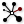Closest Point (CP)
Vector  >  Point  >  Closest Point
Find closest point in a point collection.
Inputs
Point (P)Point to search from
Cloud (C)Cloud of points to search
Outputs
Closest Point (P)Point in [C] closest to [P]
CP Index (i)Index of closest point
Distance (D)Distance between [P] and [C](i)
from the vertices at C in relation to a given point at P. Output i emits the index of the desired vertex and we can use List Item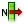List Item (Item)
Sets  >  List  >  List Item
Retrieve a specific item from a list.
Inputs
List (L)Base list
Index (i)Item index
Wrap (W)Wrap index to list bounds
Outputs
Item (i)Item at {i'}
to grab it from the list of vertices.Finding a vertex in a polyline with the smallest distance to a reference point.

### Remove vertices from a polyline

To remove a vertex from a polyline, we can deconstruct it with either Discontinuity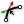Discontinuity (Disc)
Curve  >  Analysis  >  Discontinuity
Find all discontinuities along a curve.
Inputs
Curve (C)Curve to analyze
Level (L)Level of discontinuity to test for (1=C1, 2=C2, 3=Cinfinite)
Outputs
Points (P)Points at discontinuities
Parameters (t)Curve parameters at discontinuities
or Explode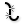Explode (Explode)
Curve  >  Util  >  Explode
Explode a curve into smaller segments.
Inputs
Curve (C)Curve to explode
Recursive (R)Recursive decomposition until all segments are atomic
Outputs
Segments (S)Exploded segments that make up the base curve
Vertices (V)Vertices of the exploded segments
and then use Cull Index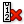Cull Index (Cull i)
Sets  >  Sequence  >  Cull Index
Cull (remove) indexed elements from a list.
Inputs
List (L)List to cull
Indices (I)Culling indices
Wrap (W)Wrap indices to list range
Outputs
List (L)Culled list
to remove a point at the specified index. Afterward, we use the remaining list to create a new PolyLine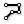PolyLine (PLine)
Curve  >  Spline  >  PolyLine
Create a polyline connecting a number of points.
Inputs
Vertices (V)Polyline vertex points
Closed (C)Close polyline
Outputs
Polyline (Pl)Resulting polyline
.

### Add vertices to a polyline

To add a vertex to a polyline, we deconstruct it first and create a list with the new and existing vertices. Then, Sort Along Curve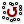Sort Along Curve (AlongCrv)
Vector  >  Point  >  Sort Along Curve
Sort points along a curve
Inputs
Points (P)Points to sort
Curve (C)Curve to sort along
Outputs
Points (P)Sorted points
Indices (I)Point index map
will sort our list of points along the old polyline. This list is then used to create a modified PolyLinePolyLine (PLine)
Curve  >  Spline  >  PolyLine
Create a polyline connecting a number of points.
Inputs
Vertices (V)Polyline vertex points
Closed (C)Close polyline
Outputs
Polyline (Pl)Resulting polyline
that includes the new vertices.Adding a vertex by adding a new point.

Another possibility, to add new vertices, is using the curve parameters of the existing polyline. Note that this approach is only possible, when the new vertices are on the existing polyline. DiscontinuityDiscontinuity (Disc)
Curve  >  Analysis  >  Discontinuity
Find all discontinuities along a curve.
Inputs
Curve (C)Curve to analyze
Level (L)Level of discontinuity to test for (1=C1, 2=C2, 3=Cinfinite)
Outputs
Points (P)Points at discontinuities
Parameters (t)Curve parameters at discontinuities
returns the existing curve parameters and we merge them in a list with new ones, which are, for example, obtained from an intersection event. This list is then sorted with Sort ListSort List (Sort)
Sets  >  List  >  Sort List
Sort a list of numeric keys.
Inputs
Keys (K)List of sortable keys
Values A (A)Optional list of values to sort synchronously
Outputs
Keys (K)Sorted keys
Values A (A)Synchronous values in A
and we compute the points by connecting the curve parameters and the initial polyline to an Evaluate Curve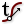Evaluate Curve (Eval)
Curve  >  Analysis  >  Evaluate Curve
Evaluate a curve at the specified parameter.
Inputs
Curve (C)Curve to evaluate
Parameter (t)Parameter on curve domain to evaluate
Outputs
Point (P)Point on the curve at {t}
Tangent (T)Tangent vector at {t}
Angle (A)Angle (in Radians) of incoming vs. outgoing curve at {t}
component. We create the new polyline with PolyLinePolyLine (PLine)
Curve  >  Spline  >  PolyLine
Create a polyline connecting a number of points.
Inputs
Vertices (V)Polyline vertex points
Closed (C)Close polyline
Outputs
Polyline (Pl)Resulting polyline
.

### Replace vertices in a polyline

To replace a vertex, we deconstruct the polyline and use Replace ItemsReplace Items (Replace)
Sets  >  List  >  Replace Items
Replace certain items in a list.
Inputs
List (L)List to modify
Item (I)Items to replace with. If no items are supplied, nulls will be inserted.
Indices (i)Replacement index for each item
Wrap (W)If true, indices will be wrapped
Outputs
List (L)List with replaced values
on the list of vertices. After modifying the desired point(s), we create a new PolyLinePolyLine (PLine)
Curve  >  Spline  >  PolyLine
Create a polyline connecting a number of points.
Inputs
Vertices (V)Polyline vertex points
Closed (C)Close polyline
Outputs
Polyline (Pl)Resulting polyline
.

For advanced, yet specific, modifications, we can also make use of the full set of methods that are described in the how-to Filter lists.

This page is open source. Edit it on GitHub or see how you can contribute.